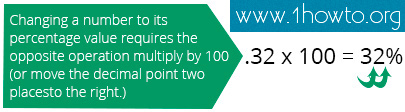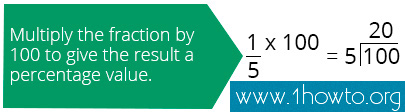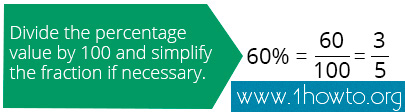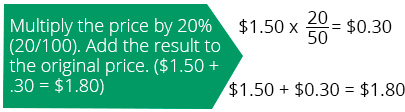﻿ How to Calculate Percentage
www.1howmany.com
Education & Reference / How to Calculate Percentage

# How to Calculate Percentage

In the modern world one cannot do without percentages1. They are used almost in every field of activity. Even when you go shopping you see advertisements for discounts of such-and-such percentages. It means that you have to know what a percentage is, and how to calculate it. In addition, you should better learn to convert decimals and fractions to percentages and vice versa, because it can be very useful in many different situations.

So, let us start from the very beginning.

The Meaning of Percentage

Percentage can be easily explained by the phrase "out of a hundred". For example, if twenty four apples out of a hundred that you had bought turned out to be rotten, you can just say that 24% of the apples were putrid. You must admit that it is more convenient and simple.

If you want to write a percentage as a decimal, you should shift the point two figures to the left, like in the picture below.And vice versa, in order to write a decimal as a percentage, move the point two figures to the right.How to Calculate Percentages

One can calculate a percentage or convert from it using very simple formulae.

Converting decimals or fractions to percentages requires multiplying by 100.And in order to convert percentages to fractions, you have to divide them by 100. Then you can reduce them if it is possible.Percentage Calculations in Everyday Life

We will give you several helpful examples of how to calculate percentages in practice.

• You sell chocolate bars. Their price is .50. You need to raise it 20%. How can you compute the new price? First, calculate one percent of 1.50 by dividing the price by 100. You will get 0.15. Then multiply 0.015 by 20 (you will get 0.3) and add the result to 1.50. The new price will be .8.• 12 pencils out of 25 are red. How can you convey it in percentage terms? Just multiply the fraction 12/25 by 100. In this case, after cancelling all you have to do is multiply 12 by 4, which means 48%.• You remember that the tax on a product that you have bought is equal to .00 but you have forgotten how much does it cost without the tax. What should you do to calculate its price? If you have a calculator ready at hand, you can just divide .00 by 15 (in order to calculate what 1% equals to) and then multiple it by 100%. An alternative way of calculating is shown in the following picture.The Universal Rule of Calculating Percentages

In most cases, you should start by counting what one percent is (dividing by 100) and then use the derived number in your further calculations. Sometimes it is more convenient to find 10% (dividing by 10).

If everything that we have explained above is too difficult for you, just use the calculator with the function of percentage calculation.
You may be interested in: